﻿ A Mathematical Model for Prediction of Metakaolin-Silica Fume High Strength ConcreteJournal of Civil Engineering Research

p-ISSN: 2163-2316    e-ISSN: 2163-2340

2017;  7(5): 137-142

doi:10.5923/j.jce.20170705.02### A Mathematical Model for Prediction of Metakaolin-Silica Fume High Strength Concrete

T. C. Nwofor, C. Ukpaka

Department of Civil Engineering, University of Port Harcourt, Nigeria

Correspondence to: T. C. Nwofor, Department of Civil Engineering, University of Port Harcourt, Nigeria.
 Email: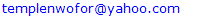Abstract

The application of mineral additives in concrete mix design for the purpose of obtaining high concrete strength was investigated in this article. A proportion of silica fume, Metakaolin, and Superplasticizier were add to conventional cement-aggregate mix in different proportions and a compressive strength of about 60N/mm2 was targeted. Multiple regression models were then applied to the experimental data and predictive models obtained for 7, 14 and 28days. The basic observation seen by introducing two kinds of mineral additives was that 28th day strength of concrete with metakaolin gave a strength of 76.04N/mm2 at 10% replacement level while silica fume produced a 28th day strength of 73.76/mm2 at the same optimum replacement level with an average error of about 3.85% estimated between the experimental and predicted data.

Keywords: Metakaolin, High strength concrete, Regression model, Compressive strength

Cite this paper: T. C. Nwofor, C. Ukpaka, A Mathematical Model for Prediction of Metakaolin-Silica Fume High Strength Concrete, Journal of Civil Engineering Research, Vol. 7 No. 5, 2017, pp. 137-142. doi: 10.5923/j.jce.20170705.02.

### 1. Introduction

The need to have high strength structural elements to carry loads especially in tall structures without necessarily increasing the size of the members has necessitated the investigation of the introduction of supplementary cementations materials sometimes called mineral additives or pozzolans. Hence it is important that concrete gains good early strength by introducing these mineral additives compared to ordinary concrete production. High strength concrete has extraordinary rheological properties and hence the development of strength is more complex in nature majorly due to the physiochemical effects of the pozzolans that would be added in most times to achieve such high strength [1-4]. Some good attributes of high strength concrete includes: high workability, high modulus of elasticity, high density, low permeability and high resistance to chemical attack . Noting that the European Standard EN 206-2000 , define high-performance concrete as specially designed concrete with one or more attributes as listed above, enhanced through the selection of component materials in adequate mix proportions aimed at producing concrete with compressive strength above 60N/mm2 at the design age of 28days. From the foregoing high-strength concrete can be made with a combination of cement which acts as a cheap binder and any mineral components such as slag, silica fume, metakaolin, fly ash, fillers e.t.c. [7, 8].
Silica fume is known to improve the mechanical property and durability of concrete because it can act as filler which fits into space between concrete grains, similar to what cement-sand paste does in the concrete mix. Hence it provides adequate packing of the concrete particles [9, 10]. Metakaolin is a profoundly effective pozzolana and responds quickly as it is produced from calcinations [11, 12]. It has the ability to supplement silica fume in areas where it is not readily available and hence becomes very costly.
The goal of this study is to develop a mathematical model based on a regression approach for prediction of compressive strength of above 60N/mm2 for the selected additives. Considering that the prediction of strength of high-strength concrete is difficult due to the complex nature of the strength gaining process, several researchers have developed models to predict the compressive strength where recent studies have related the water-cement ratio and the percentage content of the pozzolanic material present [3, 13, 14]. In this study the improvement in strength obtained by the addition of two kinds of mineral additives, silica fume and metakaolin is related and predicted through a mathematical model having in mind that most design procedures to predict strength are based on normal cement-aggregate concrete.

### 2. Materials and Method

#### 2.1. Materials

The materials to be utilized in this research include water, cement, fine and coarse aggregates and mineral pozzolans of silica fume and metakaolin. Metakaolin was obtained from Kent and Partners Limited Onitsha, while silica fume was gotten from Tohabs Engineering Company, all in Nigeria. The physical property of specific gravity of the cement, metakaolin and silica fume was found to be 3.12, 2.55 and 2.20 with an average particle size 24, 9.3, and 95.5um respectively. The coarse aggregate used in this investigation were well graded with a normrinal size of 10-20mm with a specific gravity of 2.60, while the fine aggregates which constitutes of river sand was classified in zone 2 with a specific gravity of 2.67. A summary of the chemical composition of the cementitious material is given in Table 1. A super plasticizer consisting of 20 percent solids with a specific gravity of 1.09 was used. The water used was clean at a temperature of about 29. A proper mix design procedure was carried out to produce different specimens of concrete for 72 molds of size 150 x150mm indicating a total of six molds for each specimen (three each for the 7 and 28day compressive teat). The ordinary cement was replaced by the mineral additives at 5, 10 and 15% for a constant water-cement ratio of 0.29 which is made possible through the addition of the super plasticizer. A summary of the mass proportioning is seen in Table 2.
 Table 1. Chemical Composition of Cement, Silica Fume and Metakaolin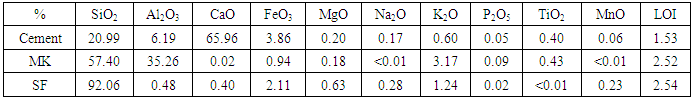Table 2. Mix Proportions for Different Specimens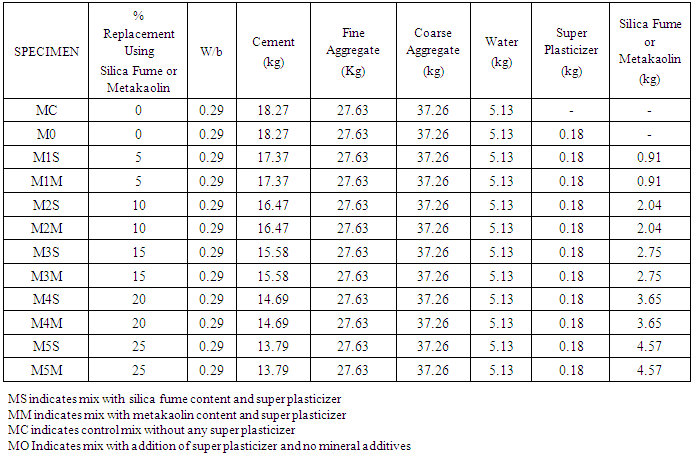#### 2.2. Mathematical Model for Simulation of Metakaolin, Silica Fume High Strength Concrete

In recent research effort is placed in developing mathematical model for prediction of strength of concrete. The common regression equation used for this purpose of simulation of compressive strength of concrete is described as follows: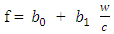(1)
Where,
f = Compressive strength of concrete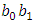= Emperical coefficients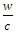= Water/Cement ratio
The application of this concept in Equation 1 is based on a linear regression model for the prediction of the compressive strength of normal concrete which is a progression from Equation 2 originally developed by Abram (1918).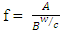(2)
Where,
f = Compressive strength of concrete; A and B are empirical coefficients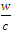= Water-cement ratio
It is common knowledge that the compressive strength of concrete decreases linearly as the water-cement (w/c) increases, hence the strength of concrete increases linearly with cement-water ratio (c/w), which modifies the Equation 2 to 3.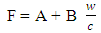(3)
For the purpose of this research, Equation 1 to 3 is modified as follows to incorporate all the constituents used in this investigation;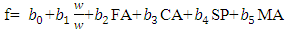(4)
Equation 4 can also be written as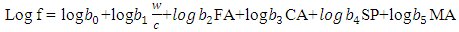(5)
Equation 5 is gotten by applying natural log to both side of Equation 4. However, Equation 5 is then reverse back to simulate the compressive strength of concrete which is the dependent variable in the modify equation to give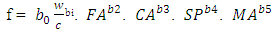(6)
Where,
FA = quantity of fine sand aggregate
CA = quantity of coarse aggregate
SP = quantity of superplasticizer
MA = quantity of mineral additive (metakaolin or silica fume)
The coefficients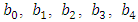and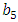stand for partial regression coefficients of f on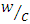, when other variables are held constant.
This research investigation established the model for compressive strength of concrete at 7, 14 and 28 days respectively in Equations 7, 8 and 9 by obtaining the adequate regression coefficients.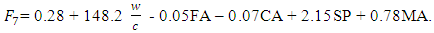(7)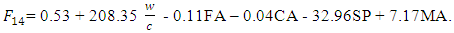(8)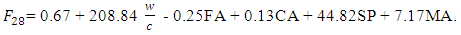(9)

### 3. Results and Discussion

Specimens were tested to determine the characteristic compressive strength of high strength concrete. The cubes were loaded in a compressive testing machine at the rate of 0.3 N/mm2/s until failure. The results are tabulated as shown in Table 3, which shows that increase in silica fume and Metakaolin content, increased the compressive strength of concrete with optimum replacement level at 10% after which the compressive strength starts to decline with further increase in replacement level.
Table 4 and 5, Figure 1, 2 and 3 gives the relationship between the laboratory determined compressive strength of concrete and the modeled compressive strength of concrete.
 Table 3. Compressive strength results for specimens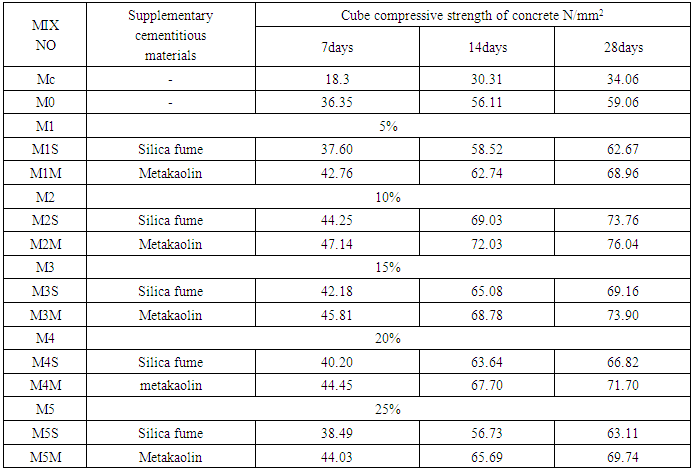Table 4. Regression coefficients for 7, 14 & 28 days for formulation of models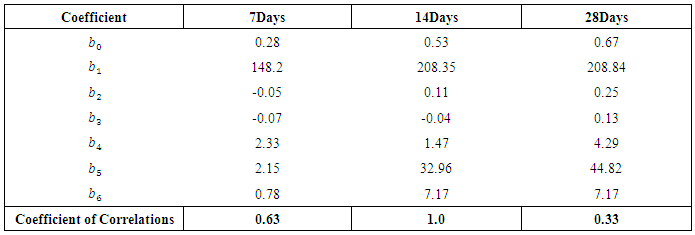Table 5. Comparison of experimental and predicted results from models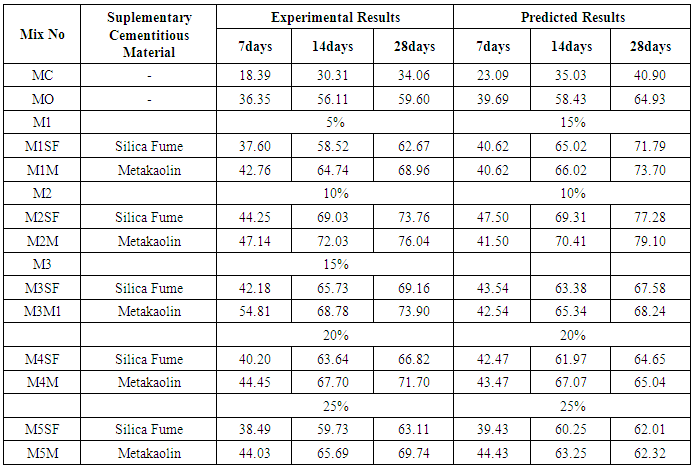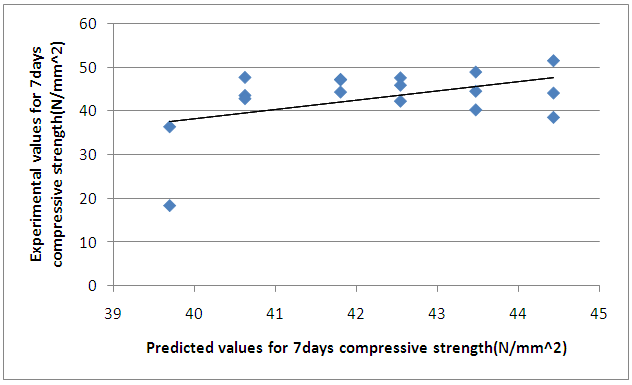Figure 1. Plot of relationship between experimental and predicted values for 7days compressive strength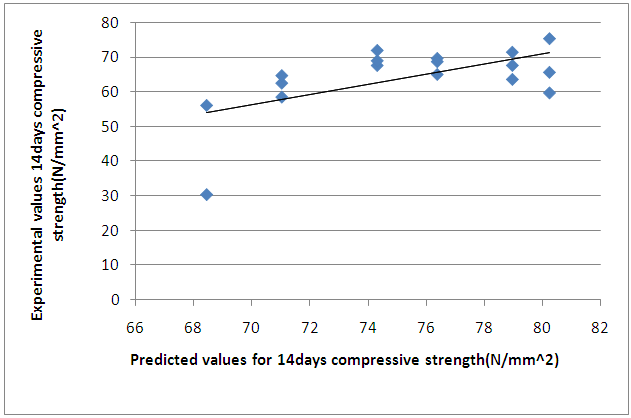Figure 2. Plot of relationship between experimental and predicted values for 14days compressive strength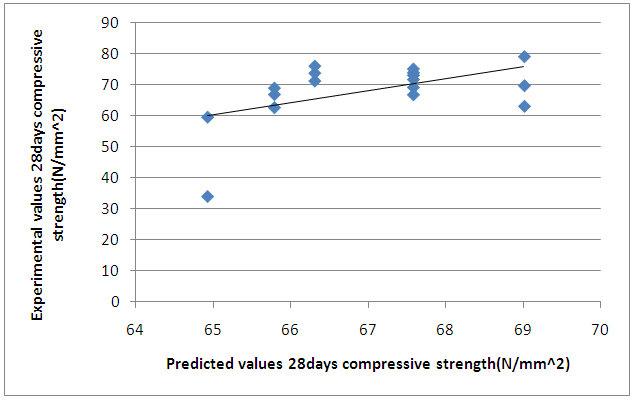Figure 3. Plot of relationship between experimental and predicted values for 28days compressive strength

### 4. Conclusions

The compressive strength results obtained in the study reflects that high concrete strength can be achieved from the inclusion of additives such as silica fume and metakaolin at a reduce water-cement ratio aided by a super plasticizer to enhance the workability of the concrete materials.
The following specific conclusions are drawn from the study.
i. The value for compressive strength observed for mixes with additives gained higher 7th day strength.
ii. The 28th day strength of concrete with metakaolin gave a strength of 76.04N/mm2 at 10% replacement level while silica fume produced a 28th day strength of 73.76/mm2 at the same optimum replacement level.
iii. The average error between the experimental and predicted data was about 3.85%.

### References

  Aitein, P.C. (2000). Cement yesterday and today. Concrete of tomorrow. Cement and Concrete Research 30, 1349-1359.  Aitein, P.C. (1998). High performance concrete. London: E & FN SPON.  Kapelko, A., Kapelko, M. & Kapelko, R. (2010). Modeling compressive strength of high-performance concrete with multiple regression. Advances and Trends in Structural Engineering, Mechanics and Computation, London, Taylor & Francis Group.  Zain, M.F.M., Safiuddin, M. & Yusof, K.M. (1999). A Study on the properties of freshly mixed high performance concrete. Concrete and Concrete Research 29, 1427-1432.  Neville, A. & Aitein, P.C. (1998). High performance concrete-an overview. Materials and Structures 31, 111-117.  EN 206-1:2000 Concrete-Part 1: Specification, performance, production and conformity. Brussels, 2000.  Aitein, P.C. (2003). The durability characteristics of high-performance concrete: a review. Cement and Concrete Research 25, 409-420.  Dinakar, P., Pradosh, K.S. and Sriram, G. (2013). Effects of metakaolin content on the properties of high strength concrete. International Journal of Concrete Structures and Materials, 7(3), 215-223.  Basu, P. C. (2003). High performance concrete. In Proceedings INAE national seminar on engineered building materials and their performance, 426–450.  Abdul, R. H., & Wong, H. S. (2005). Strength estimation model for high-strength concrete incorporating metakaolin and silica fume. Cement Concrete Research, 35(4), 688–695.  Basu, P. C., Mavinkurve, S., Bhattacharjee, K. N., Deshpande, Y., & Basu, S. (2000). High reactivity metakaolin: A supplementary cementitious material. In Proceedings ICIAsian conference on ecstasy in concrete, 20–22 Nov, Bangalore, India, 237–436.  Tiwari, A. K., & Bandyopadhyay, P. (2003). High performance concrete with Indian metakaolin. In International symposium on innovative world of concrete, 19–21 September. Pune: Indian Concrete Institute.  Popovics, S. & Ujhelyi, J. (2008). Contribution to the concrete strength versus water-cement ratio relationship. Journal of Materials in Civil Engineering 20(7): 459-463.  Zain, M.F.M. & Abd, S.M. (2009). Multiple regression model for compressive strength prediction of high performance concrete. Journal of Applied Sciences 9(1): 155-160.